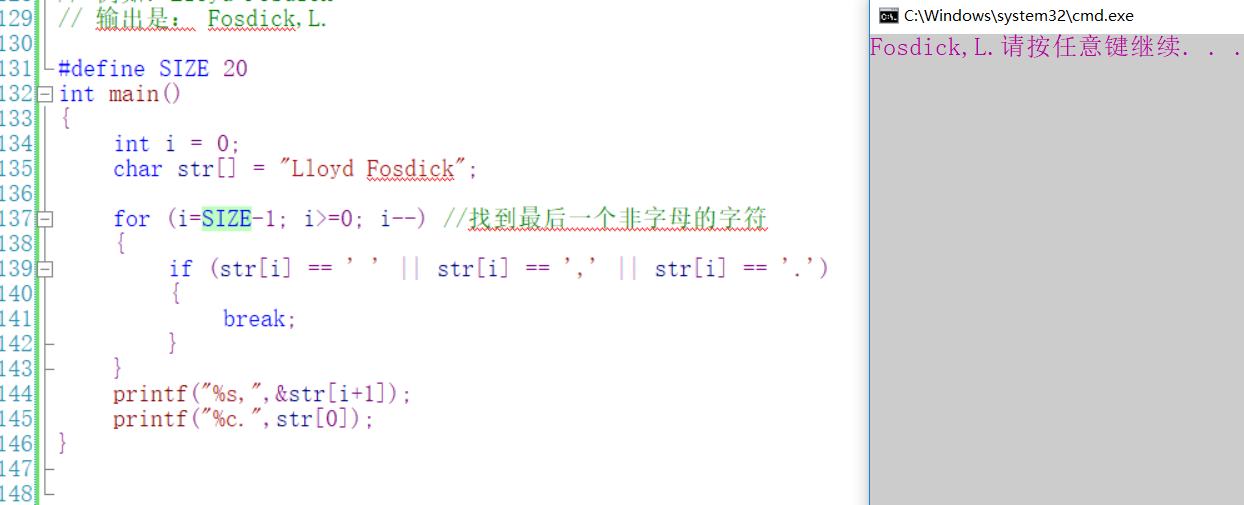c语言编写一个程序，根据用户输入英文名和姓先显示姓氏，其后跟一个逗号，然后显示名的首字母 10C

c语言编写一个程序，根据用户输入英文名和姓先显示姓氏，其后跟一个逗号，然后显示名的首字母。

10个回答u011029018 如果是c语言，那就遍历字符串找到空格的位置，然后进行字符串的切片操作，如果是c++，直接有函数split进行操作。 在分割好后，先输出后面的字符串，再输出“,”，最后再输出字符串的第一个字母。whyyouhitme_ 回复Siege-lion: 老哥。如果用户的输入里没有空格呢？u011029018 如果是c语言，那就遍历字符串找到空格的位置，然后进行字符串的切片操作，如果是c++，直接有函数split进行操作。 在分割好后，先输出后面的字符串，再输出“,”，最后再输出字符串的第一个字母。whyyouhitme_ 回复GHH120338: 老哥，请注意题目。说是有可能会加空格。也就是，也有可能不加空格。所以我是按大写字母区分的。

# include

int main()
{
char ch;
char chf;
char chl;
int i=0, j=0, k=0;
//录入字符串
while(scanf("%c", &ch) && ch!='\n')
{
chl[i]=ch;
i++;
}
//求前面的空格
for (int c=0;c if (chl[c]>=65 && chl[c]<=90)
break;

``````//去空格
for (int d=0;d<c;d++)
for (int e=c-1;e<i;e++)
chl[e-1]=chl[e];
i=i-c;

//找姓的首字母地址
for (j=1;j<i;j++)
if (chl[j]>=65 && chl[j]<=90)
break;

//录入姓
while (j<i)
{
chf[k]=chl[j];
k++;
j++;
}
//输出
for (int a=0; a<k; a++)
{
printf ("%c", chf[a]);
if (chf[a+1]==' ')
break;
}

printf (",");
printf ("%c",chl);
return 0;
``````

}

``````
``````#include
#include
int main(int argc, char **argv)
{
char s_name;
char f_name;
char l_name;
int i=0;

``````    printf("please enter your name!\n");
fgets(s_name, 256, stdin);
printf("%s\n",s_name);
strncpy(f_name, s_name, 1);
printf("%s\n", f_name);
for (i=1; s_name[i] != '\0'; i++)
{
if (s_name[i] >= 'A' && s_name[i] <= 'Z')
{
break;
}
}
strncpy(l_name, s_name+i, strlen(s_name)-i-1);

printf("%s,%s\n", l_name, f_name);

return 0;
``````

}

``````
``````Vist_1 复制的有问题，前两行是#include <stdio.h>和#include <string.h>

``````  我的想法是:按老外的习惯，他们的首字母会大写，弄俩字符串，分别记录名和姓，遇到大写，就是65到106开始，把名输到名的字符串里面，遇到第二个大写输到姓的字符串里面，然后输出。
``````

``````#define SIZE 20
int main()
{
int i = 0;
char str[] = "Lloyd Fosdick";

for (i=SIZE-1; i>=0; i--) //找到最后一个非字母的字符
{
if (str[i] == ' ' || str[i] == ',' || str[i] == '.')
{
break;
}
}
printf("%s,",&str[i+1]);
printf("%c.",str);
}

``````/*去除字符串右边空格*/

void StrRTrim(char *pStr)

{

char *pTmp = pStr+strlen(pStr)-1;

``````while (*pTmp == ' ')
{
*pTmp = '\0';
pTmp--;
}
``````

}

/*去除字符串左边空格*/

void StrLTrim(char *pStr)

{

char *pTmp = pStr;

``````while (*pTmp == ' ')
{
pTmp++;
}
while(*pTmp != '\0')
{
*pStr = *pTmp;
pStr++;
pTmp++;
}
*pStr = '\0';
``````

}

int StrName(char *pStr, int nLen)
{
int i = 0;

``````for (i=nLen-1; i>=0; i--) //找到最后一个非字母的字符
{
if (pStr[i] == ' ')
{
break;
}
}
printf("%s,",pStr + i);
printf("%c.", *pStr);
return 0;
``````

}

void main()
{
char str[] = " Lloyd Fosdick ";
StrLTrim(str);
StrRTrim(str);
StrName(str, strlen(str));
}